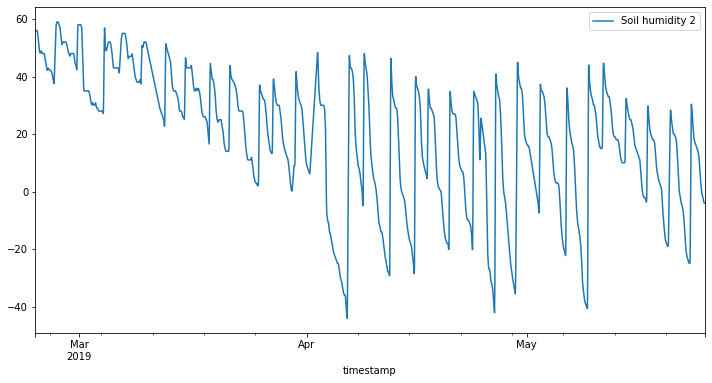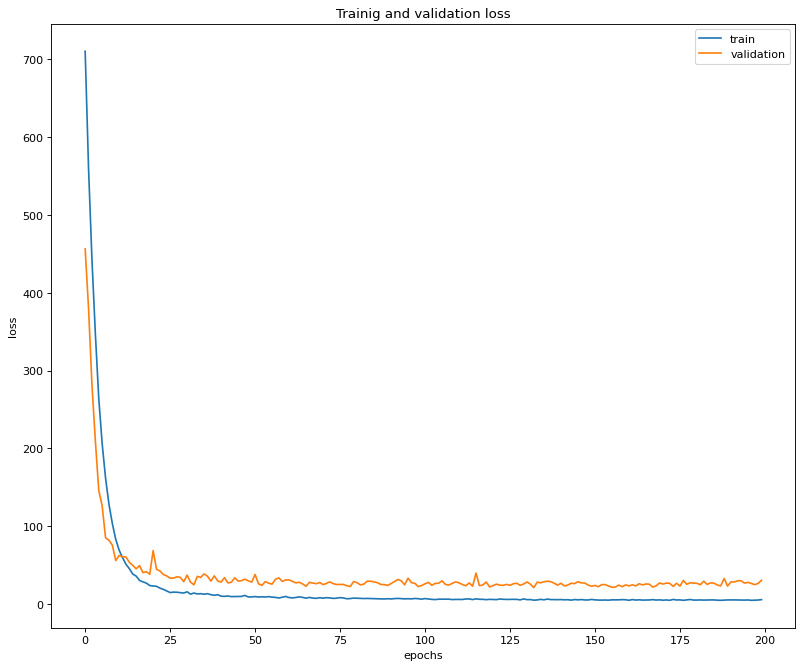# Forecasting soil moisture levels using Long Short-Term Memory (LSTM) model

Time series analysis can help farmers understand the dynamic state of their farms, thus empowering them to make quicker and smarter decisions.

Author: Neha PokharelTime series analysis and prediction is an important component of Project Saathi. Our group, Insight, focuses on time series analysis of various important parameters of the soil. We want to help the farmers make the right decisions for their farms by analyzing soil data from the sensors, ultimately helping them understand the dynamic state of their farms and empowering them to make quicker and smarter decisions. Although there are numerous techniques that can be used to evaluate time series data, this experiment and specifically this article focuses on using a univariate LSTM model to understand and forecast time series for soil moisture levels.

1. Data
The moisture data used in this experiment is from zindi and contains 28049 number of data points (later cleaned, which included removal of NaN values). The dataset consists of various parameters but we will only require the ‘moisture’ column for this experiment. The final time series moisture dataset looks like the following:2. Splitting the data

The data is extracted and split into training and test datasets in a 90:10 ratio. These split datasets are subsequently converted into lists.

``````train_size = int(moisture_df.shape*0.90)
train_df = moisture_df.iloc[:train_size]
test_df = moisture_df.iloc[train_size:]

listed_train = train_df["Moisture"].to_list()
listed_test = test_df["Moisture"].to_list()``````

The dataset is further chunked into smaller blocks based on the number of inputs and outputs required for the model.

``````def create_dataset(x, lookback_steps, number_of_predictions):
'''splits the data into x and y
Args:
x: input dataset
lookback_steps: number of inputs for the model
number_of_predictions: number of output data points
Returns:
X and y
'''
Xs, ys = [], []
for i in range(len(x)):
# find the end of this pattern
end_ix = i + lookback_steps
out_end_ix = end_ix + number_of_predictions
# check if we are beyond the sequence
if out_end_ix > len(x):
break
# gather input and output parts of the pattern
seq_x, seq_y = x[i:end_ix], x[end_ix:out_end_ix]
Xs.append(seq_x)
ys.append(seq_y)
return np.array(Xs), np.array(ys)``````

In this particular example we are using the following configuration to call this function:

``````number_of_predictions = 2
lookback_steps = 100
X_train, y_train = create_dataset(listed_train,lookback_period, number_of_predictions)``````

Here, the lookback steps is 100 and the number of predictions is 2.

3. Building and Fitting the Model

The datasets we have created are used in a sequential model that consists of  multiple layers including Conv1D, LSTM, Dropout, Dense. The unit of the Dense layer determines the number of outputs, which is 2 for this particular scenario.

``````model = Sequential()

This model is fitted with a batch size of 32 and a validation split of 10 percent is used to evaluate the performance of the model on every epoch.

``model.fit(X_train, y_train, epochs=200, batch_size=32, validation_split = 0.1)``

A 200 epoch run resulted in the following training and validation losses:4. Batch Forecasting

While implementing this model to forecast the moisture levels, a latest data sample of the size `lookback_steps` is used to generate new forecasts. In this particular example, 100 data points are used to forecast 2 new data points.

However, a practical use case generally would require a larger forecast dataset. To solve this the newly generated data points (in this case 2) are appended to the original dataset. By doing this we have now created a dataset which contains new data points on the tail of the original dataset. Taking the latest `lookback_steps` now generates completely new forecasts. These steps are repeated until we obtain the desired number of forecast points.

In this particular experiment, Batch Forecasting was used to forecast 50 new data points in 25 prediction iterations:The data points after the blue line are completely new data points forecasted by the model. As we can observe from the graph, in general the forecasted dataset looks promising. The model is able to maintain reasonable forecasts with a root mean square error of 8.0. It starts to deviate slowly when it has to forecast bigger datasets using increasingly smaller known datasets, which is quite acceptable.